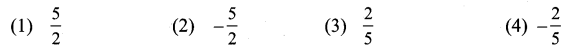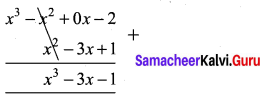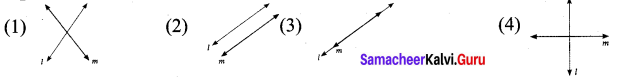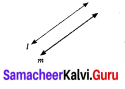# Samacheer Kalvi 9th Maths Solutions Chapter 3 Algebra Ex 3.15

## Tamilnadu Samacheer Kalvi 9th Maths Solutions Chapter 3 Algebra Ex 3.15

MULTIPLE CHOICE QUESTIONS :
Question 1.
If x3 + 6x2 + kx + 6 is exactly divisible by (x + 2), then k = ?
(1) -6
(2) -7
(3) -8
(4) 11
Solution:
(4) 11
Hint: P(-2) = (-2)3 + 6 (-2)2 + k (-2) + 6 = 0
⇒ – 8 + 24 – 2k +6 = 0
⇒ 22 = 2k
⇒ k = 11

Question 2.
The root of the polynomial equation 2x + 3 = 0 is
(1) $$\frac{1}{3}$$
(2) $$-\frac{1}{3}$$
(3) $$-\frac{3}{2}$$
(4) $$-\frac{2}{3}$$
Solution:
(3) $$-\frac{3}{2}$$

Question 3.
The type of the polynomial 4 – 3x3 is
(1) constant polynomial
(2) linear polynomial
(4) cubic polynomial.
Solution:
(4) cubic polynomial.
Hint: Polynomial of degree 3 is called cubic.Question 4.
If x51 + 51 is divided by x + 1, then the remainder is
(1) 0
(2) 1
(3) 49
(4) 50
Solution:
(4) 50
Hint: P(-1 = (-1)51 + 51 = -1 + 51 = 50

Question 5.
The zero of the polynomial 2x + 5 isSolution:
(2) $$-\frac{5}{2}$$

Question 6.
The sum of the polynomials p(x) = x3 – x2 – 2, q(x) = x2 – 3x + 1
(1) x3 – 3x – 1
(2) x3 + 2x2 – 1
(3) x3 – 2x2 – 3x
(4) x3 – 2x2 + 3x – 1
Solution:
(1) x3 – 3x – 1
Hint:Question 7.
Degree of the polynomial (y3 – 2) (y3 + 1) is
(1) 9
(2) 2
(3) 3
(4) 6
Solution:
(4) 6
Hint: (y3 – 2)(y3 + 1) = (y3 – 2)(y3 – 2) × 1 = y6 – 2y3 + y3 – 2 = y6 – y3 – 2Question 8.
Let the polynomials be
(A) -13q5 + 4q2 + 12q
(B) (x2 + 4 ) (x2 + 9)
(C) 4q8 – q6 + q2
(D) $$-\frac{5}{7}$$y12 + y3 + y5
Then ascending order of their degree is
(1) A, B, D, C
(2) A, B, C, D
(3) B, C, D, A
(4) B, A, C, D
Solution:
(4) B, A, C, D
Hint: Degree of (A), (B) (C) & (D) are respectively be 5, 4, 8, 12

Question 9.
If p (a) = 0 then (x – a) is a ________ of p(x)
(1) divisor
(2) quotient
(3) remainder
(4) factor
Solution:
(4) factor

Question 10.
Zeros of (2 – 3x) is _____
(1) 3
(2) 2
(3) $$\frac{2}{3}$$
(4) $$\frac{3}{2}$$
Solution:
(3) $$\frac{2}{3}$$
Hint: 2 – 3x =0
-3x = – 2
x = $$\frac{2}{3}$$

Question 11.
Which of the following has x – 1 as a factor?
(1) 2x – 1
(2) 3x – 3
(3) 4x – 3
(4) 3x – 4
Solution:
(2) 3x – 3
Hint: p(x) = 3x – 3
P( 1) = 3(1) – 3 = 0
∴ (x – 1) is a factor of p(x)

Question 12.
If x – 3 is a factor of p (x), then the remainder is
(1) 3
(2) -3
(3) p(3)
(4) p(-3)
Solution:
(3) p(3)Question 13.
(x + y)(x2 – xy + y2) is equal to
(1) (x + y)3
(2) (x – y)3
(3) x3 + y3
(4) x3 – y3
Solution:
(3) x3 + y3

Question 14.
(a + b – c)2 is equal to
(1) (a – b + c)2
(2) (-a – b + c)2
(3) (a + b + c)2
(4) (a – b – c)2
Solution:
(2) (-a – b + c)2
Hint: (a + b – c)2 = [- (- a – b + c)]2 = (-a – b + c)2

Question 15.
In an expression ax2 + bx + c the sum and product of the factors respectively,
(1) a, bc
(2) b, ac
(3) ac, b
(4) bc, a
Solution:
(2) b, ac

Question 16.
If (x + 5) and (x – 3) are the factors of ax2 + bx + c, then values of a, b and c are
(1) 1, 2, 3
(2) 1, 2, 15
(3) 1, 2, -15
(4) 1, -2, 15
Solution:
(3) 1, 2, -15
Hint: p(-5) = a (-52) + b (-5) + c = 25a – 5b + c = 0 ……… (1)
p( 3) = a (32) + bc + 3 + c = 9 + 3b + c = 0 …….. (2)
25a – 5b = 9a + 3b
25a – 9a = 3b + 5b
16a = 8 b
$$\frac{a}{b}=\frac{8}{16}=\frac{1}{2}$$
Substitute a = 1,b = 2 in (1)
25(1) – 5 (2) = -c
25 – 10 = 15 = – c
c = -15

Question 17.
Cubic polynomial may have a maximum of
(1) 1
(2) 2
(3) 3
(4) 4
Solution:
(3) 3

Question 18.
Degree of the constant polynomial is
(1) 3
(2) 2
(3) 1
(4) 0
Solution:
(4) 0

Question 19.
Find the value of m from the equation 2x + 3y = m. If its one solution is x = 2 and y = – 2.
(1) 2
(2) -2
(3) 10
(4) 0
Solution:
(2) -2
Hint: 2x + 3y = m, x = 2, y = – 2
m = 2(2) + 3(-2)
= 4 – 6 = -2

Question 20.
Which of the following is a linear equation?
(1) x + $$\frac{1}{x}$$ = 2 x
(2) x(x – 1) = 2
(3) 3x + 5 = $$\frac{2}{3}$$
(4) x3 – x = 5
Solution:
(3) 3x + 5 = $$\frac{2}{3}$$
Hint: x + $$\frac{1}{x}$$ = 2 ⇒ x2 – 2x + 1 = 0; x(x – 1) = 2 ⇒ x2 -x – 2 = 0

Question 21.
Which of the following is a solution of the equation 2x – y = 6?
(1) (2, 4)
(2) (4, 2)
(3) (3, -1)
(4) (0, 6)
Solution:
(2) (4, 2)
Hint: 2x – y = 6
2(4) – 2 = 8 – 2 = 6 = RHS

Question 22.
If (2, 3) is a solution of linear equation 2x + 3y = k then, the value of k is
(1) 12
(2) 6
(3) 0
(4) 13
Solution:
(4) 13
Hint: 2x + 3y = k
2(2) + 3(3) = 4 + 9 = 13Question 23.
Which condition does not satisfy the linear equation ax + by + c = 0
(1) a ≠ 0,b = 0
(2) a = 0, b ≠ 0
(3) a = 0, b = 0, c ≠ 0
(4) a ≠ 0, b ≠ 0
Solution:
(3) a = 0, b = 0, c ≠ 0
Hint: a = 0, b =0, c ≠ 0 ⇒ (0)x + (0) y + c = 0 False

Question 24.
Which of the following is not a linear equation in two variable
(1) ax + by + c = 0
(2) 0x + 0y + c = 0
(3) 0x + by + c = 0
(4) ax + 0y + c = 0
Solution:
(2) 0x + 0y + c = 0
Hint: a and b both can not be zero

Question 25.
The value of k for which the pair of linear equations 4x + 6y – 1 = 0 and 2x + ky – 7 = 0 represents parallel lines is
(1) k = 3
(2) k = 2
(3) k = 4
(4) k = -3
Solution:
(1) k = 3
Hint: 4x + 6y = 1
6y = -4x + 1
y = $$\frac{-4}{6} x+\frac{1}{6}$$ ……….. (1)
2x + ky – 7 = 0
ky = -2x + 7
y = $$\frac{-2}{k} x+\frac{7}{k}$$ ……….. (2)
Since the lines (1) and (2) parallel m1 = m2
$$\frac{-4}{6}=\frac{-2}{k}$$
k = -2 × $$\frac{-6}{4}$$ = 3

Question 26.
A pair of linear equations has no solution then the graphical representation isSolution:
(2)Hint: Parallel lines have no solution

Question 27.
If $$\frac{a_{1}}{a_{2}} \neq \frac{b_{1}}{b_{2}}$$ where a1x + b1y + c1 = 0 and a2x + b2y + c2 = 0 then the given pair of linear equation has ______ solution(s)
(1) no solution
(2) two solutions
(3) unique
(4) infinite
Solution:
(3) unique
Hint: $$\frac{a_{1}}{a_{2}} \neq \frac{b_{1}}{b_{2}}$$; unique solution

Question 28.
If $$\frac{a_{1}}{a_{2}}=\frac{b_{1}}{b_{2}} \neq \frac{c_{1}}{c_{2}}$$ where a1x + b1y + c1 = 0 and a2x + b2y + c2 = 0 then the given pair of linear equation has
(1) no solution
(2) two solutions
(3) infinite
(4) unique
Solution:
(1) no solution
Hint: $$\frac{a_{1}}{a_{2}}=\frac{b_{1}}{b_{2}} \neq \frac{c_{1}}{c_{2}}$$: parallelQuestion 29.
GCD of any two prime numbers is _______
(1) -1
(2) 0
(3) 1
(4) 2
Solution:
(3) 1

Question 30.
The GCD of x4 – y4 and x2 – y2 is
(1) x4 – y4
(2) x2 – y2
(3) (x + y)2
(4) (x + y)4
Solution:
(2) x2 – y2
Hint:
x4 – y4 = (x2 )2 – (y2)2 = (x2 + y2) (x2 – y2)
x2 – y2 = x2 – y2
G.C.D. is = x2 – y2